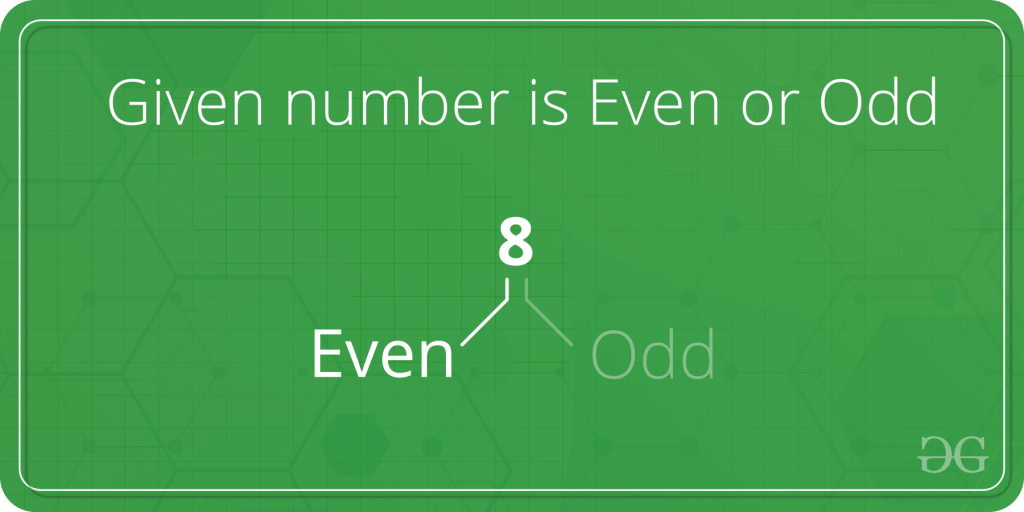# Check whether a given number is even or odd

Given a number, check whether it is even or odd.Examples :

```Input: 2
Output: even

Input: 5
Output: odd
```

## Recommended: Please solve it on “PRACTICE ” first, before moving on to the solution.

One simple solution is to find remainder after dividing by 2.

## C++

 `// A simple C++ program to  ` `// check for even or odd ` `#include ` `using` `namespace` `std; ` ` `  `// Returns true if n is  ` `// even, else odd ` `bool` `isEven(``int` `n) ` `{ ` `return` `(n % 2 == 0); ` `} ` ` `  `// Driver code ` `int` `main() ` `{  ` `int` `n = 101; ` `isEven(n)? cout << ``"Even"` `: ` `           ``cout << ``"Odd"``; ` ` `  `return` `0; ` `}  `

## Java

 `// Java program program to  ` `// check for even or odd ` ` `  `class` `GFG ` `{ ` `    ``// Returns true if n is even, else odd ` `    ``public` `static` `boolean` `isEven(``int` `n) ` `    ``{ ` `        ``return` `(n % ``2` `== ``0``); ` `    ``} ` `     `  `    ``// Driver code ` `    ``public` `static` `void` `main(String[] args) ` `    ``{ ` `        ``int` `n = ``101``; ` `        ``if``(isEven(n) == ``true``) ` `            ``System.out.print(``"Even"``); ` `        ``else` `            ``System.out.print(``"Odd"``); ` `    ``} ` `} ` ` `  `// This code is contributed by rishabh_jain `

## Python3

 `# A simple Python3 code  ` `# to check for even or odd ` ` `  `# Returns true if n is even, else odd ` `def` `isEven(n): ` `    ``return` `(n ``%` `2` `=``=` `0``) ` `     `  `# Driver code ` `n ``=` `101` `print``(``"Even"` `if` `isEven(n) ``else` `"Odd"``) ` ` `  `# This code is contributed by "Sharad_Bhardwaj". `

## C#

 `// C# program program to  ` `// check for even or odd ` `using` `System; ` ` `  `class` `GFG ` `{ ` `    ``// Returns true if n is even, else odd ` `    ``public` `static` `bool` `isEven(``int` `n) ` `    ``{ ` `        ``return` `(n % 2 == 0); ` `    ``} ` `     `  `    ``// Driver code ` `    ``public` `static` `void` `Main() ` `    ``{ ` `        ``int` `n = 101; ` `        ``if``(isEven(n) == ``true``) ` `            ``Console.WriteLine(``"Even"``); ` `        ``else` `            ``Console.WriteLine(``"Odd"``); ` `    ``} ` `} ` ` `  `// This code is contributed by vt_m `

## PHP

 ` `

Output :

```Odd
```

A better solution is to use bitwise operators. We need to check whether last bit is 1 or not. If last bit is 1 then number is odd, otherwise always even.

Explanation:

``` input : 5    // odd
00000101
& 00000001
--------------
00000001
--------------

input : 8     //even
00001000
& 00000001
--------------
00000000
--------------
```

Below is the implementation of the idea.

## C++

 `// A simple C++ program to  ` `// check for even or odd ` `#include ` `using` `namespace` `std; ` ` `  `// Returns true if n is ` `// even, else odd ` `bool` `isEven(``int` `n) ` `{ ` `     `  `// n & 1 is 1, then  ` `// odd, else even ` `return` `(!(n & 1)); ` `} ` ` `  `// Driver code ` `int` `main() ` `{  ` `int` `n = 101; ` `isEven(n)? cout << ``"Even"` `: ` `           ``cout << ``"Odd"``; ` ` `  `return` `0; ` `}  `

## Java

 `// Java program program to  ` `// check for even or odd ` ` `  `class` `GFG ` `{ ` `    ``// Returns true if n  ` `    ``// is even, else odd ` `    ``public` `static` `boolean` `isEven(``int` `n) ` `    ``{ ` `        ``if``((n & ``1``) == ``0``) ` `            ``return` `true``; ` `        ``else` `            ``return` `false``; ` `    ``} ` `     `  `    ``// Driver code ` `    ``public` `static` `void` `main(String[] args) ` `    ``{ ` `        ``int` `n = ``101``; ` `        ``if``(isEven(n) == ``true``) ` `            ``System.out.print(``"Even"``); ` `        ``else` `            ``System.out.print(``"Odd"``); ` `    ``} ` `} ` ` `  `// This code is contributed by rishabh_jain `

## Python3

 `# A Python3 code program  ` `# to check for even or odd ` ` `  `# Returns true if n is even, else odd ` `def` `isEven(n): ` `     `  `    ``# n&1 is 1, then odd, else even ` `    ``return` `(``not``(n & ``1``)) ` `     `  `# Driver code ` `n ``=` `101``; ` `print``(``"Even"` `if` `isEven(n) ``else` `"Odd"``) ` ` `  `# This code is contributed by "Sharad_Bhardwaj". `

## C#

 `// C# program program to  ` `// check for even or odd ` `using` `System; ` ` `  `class` `GFG ` `{ ` `    ``// Returns true if n  ` `    ``// is even, else odd ` `    ``public` `static` `bool` `isEven(``int` `n) ` `    ``{ ` `        ``if``((n & 1) == 0) ` `            ``return` `true``; ` `        ``else` `            ``return` `false``; ` `    ``} ` `     `  `    ``// Driver code ` `    ``public` `static` `void` `Main() ` `    ``{ ` `        ``int` `n = 101; ` `        ``if``(isEven(n) == ``true``) ` `            ``Console.WriteLine(``"Even"``); ` `        ``else` `            ``Console.WriteLine(``"Odd"``); ` `    ``} ` `} ` ` `  `// This code is contributed by vt_m. `

## PHP

 ` `

Output :

```Odd
```

This article is contributed by Prabhat Raushan. If you like GeeksforGeeks and would like to contribute, you can also write an article using contribute.geeksforgeeks.org or mail your article to contribute@geeksforgeeks.org. See your article appearing on the GeeksforGeeks main page and help other Geeks.